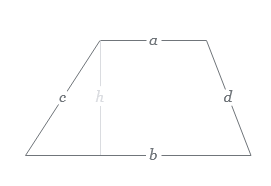Trapezoid homework Help at TutorEye

## Top Questions

t schedule 3 people for the day my % scheduled is 78.57% My question is how did the computer come up with the 78.57%?
View More

I would love to get a written solution. I did the other proof tree that you can see in the attaached files. So its supposed to look like that Thank You.
View More

xtbook. i really need in in half an hour
View More

## Trapezoid:

Trapezoid is a type of quadrilateral having one set of parallel sides and other sets of non-parallel sides.Where, ‘a’ and ‘b’ are parallel sides and ‘c’ and ‘d’ are non-parallel sides and ‘h’ is its height.

Question 1: How to find the area of a trapezoid?

The area of a trapezoid is computed by multiplying the average of the parallel sides by the height of the trapezoid.

A = 1/2 x (a + b) x heightExample:

Calculate the area of a trapezoid whose height is 15cm and length of parallel sides are 8cm and 18cm?

Area of trapezoid,

A = 1/2 x (a + b) x height

height,h=15cm

Parallel sides= 8cm and 18cm

So, Area of trapezoid = (1/2 )*15*(8+18) sq.cm

= 195 sq.cm

Question 2: What is a trapezoid?

Trapezoid is a type of quadrilateral having one set of parallel sides and other sets of non-parallel sides.Where, ‘a’ and ‘b’ are parallel sides and ‘c’ and ‘d’ are non-parallel sides and ‘h’ is its height.

There are three type of trapezoid:

• Isosceles Trapezoid: one in which the legs or non-parallel sides of the trapezoid are of equal length.
• Scalene Trapezoid: one in which neither the sides nor the angles of the trapezoid are equal.
• Right Trapezoid: one that has two right angles.